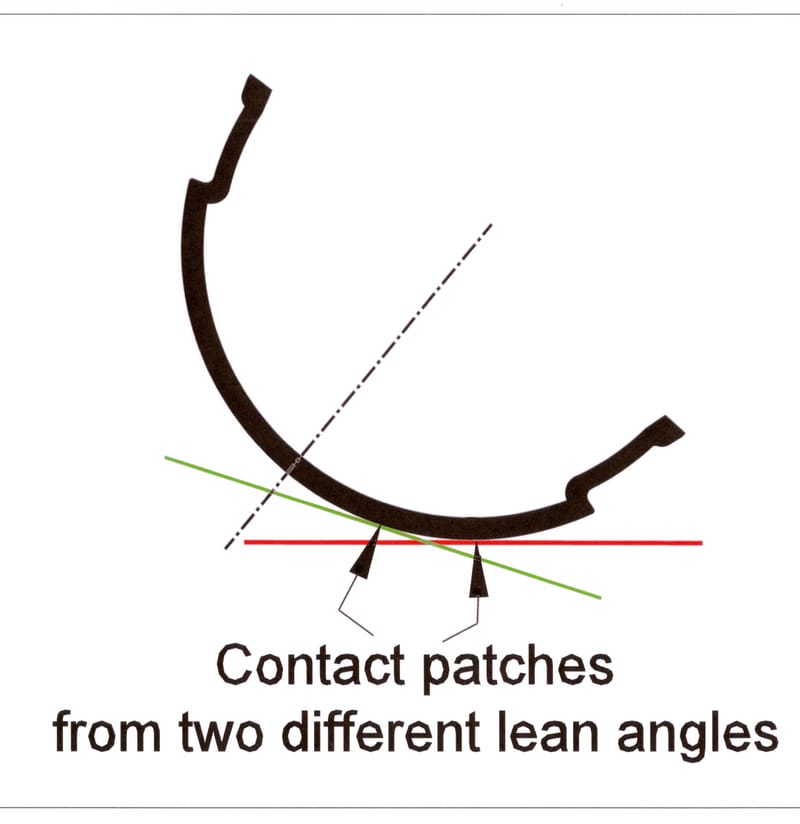# Formula for frictionThe formula for friction (f) or what we call traction is

=Mu x n

Where

F = friction

Mu = Coefficient of friction (Cf)

n = normal down force = weight or mass.

Notice that the size of the surface area is not in this formula.

Cf is determined by the two materials rubbing together such as the tire & the pavement, its unit of measurement is g. For street tires this value could be anywhere from 0.80g to 1.50g on good dry pavement. A good race and good racing pavement can develop a Cf of 1.50g or more. The normal down force is the weight or the mass of the bike on the tire.

F = Cf x weight or again F =Mu x n

To explain the changes in friction with only a change in area we will be using round numbers.

We will look at friction using a fixed weight and the known surface area.

lbs / Sq in = psi. If you have one hundred pounds on one square inch of rubber then you have

100lbs/1sq in = 100psi

100 x 1 =100 lbs on total contact patch

But, if you take the same one hundred pounds and put it on two square inches of rubber then you have

100lbs /2sq in = 50psi

50 x 2 = 100 lbs on total contact patch

You have twice the surface area but, the same total weight on the contact patch.  This results in the same friction level.

Net sum gain = zero!

So in conclusion increasing the surface area of a tire in and of itself will not increase the friction level.

To find the actual friction we will use a CF of 1.5g with 100lbs,

F= Mu x n

F=1.5g x 100lbs= 150 lbs

This means it will take 150 lbs of lateral force to cause the contact patch to slide.  This translates into a cornering force of 1.5g.

The size of the tire contact surface area will come into play when you start to calculate in other engineering principles such as thermal dynamics, mechanical stresses on the contact patch. If the surface area is too small for the mass or power of the bike, the tire will mechanically tear or over heat or shred resulting in premature and excessive tire spin or slide. If the contact area is too big then the compound will not be able to come to the correct operating temperature and not develop the max coefficient of friction and result in premature and excessive tire spin or slide.

In conclusion

The right size tire will give you the best performance. Over-sizing or under-sizing can have a negative effect on the performance of the tire. But a small change in the size, from a change in BP, if any at all, will have no effect on traction available.

The common theory about changes in contact patch size, from changes in body position alone, will be discussed in another article.